Math Olympiad Test: Area of Parallelograms and Triangles- 2

# Math Olympiad Test: Area of Parallelograms and Triangles- 2

Test Description

## 15 Questions MCQ Test Mathematics Olympiad for Class 9 | Math Olympiad Test: Area of Parallelograms and Triangles- 2

Math Olympiad Test: Area of Parallelograms and Triangles- 2 for Class 9 2022 is part of Mathematics Olympiad for Class 9 preparation. The Math Olympiad Test: Area of Parallelograms and Triangles- 2 questions and answers have been prepared according to the Class 9 exam syllabus.The Math Olympiad Test: Area of Parallelograms and Triangles- 2 MCQs are made for Class 9 2022 Exam. Find important definitions, questions, notes, meanings, examples, exercises, MCQs and online tests for Math Olympiad Test: Area of Parallelograms and Triangles- 2 below.
Solutions of Math Olympiad Test: Area of Parallelograms and Triangles- 2 questions in English are available as part of our Mathematics Olympiad for Class 9 for Class 9 & Math Olympiad Test: Area of Parallelograms and Triangles- 2 solutions in Hindi for Mathematics Olympiad for Class 9 course. Download more important topics, notes, lectures and mock test series for Class 9 Exam by signing up for free. Attempt Math Olympiad Test: Area of Parallelograms and Triangles- 2 | 15 questions in 15 minutes | Mock test for Class 9 preparation | Free important questions MCQ to study Mathematics Olympiad for Class 9 for Class 9 Exam | Download free PDF with solutions
 1 Crore+ students have signed up on EduRev. Have you?
Math Olympiad Test: Area of Parallelograms and Triangles- 2 - Question 1

### If a triangle and a parallelogram are on the same base and between the same parallels, and area of triangle is A, then area of parallelogram is:

Detailed Solution for Math Olympiad Test: Area of Parallelograms and Triangles- 2 - Question 1

Area of ∆ = 1/2 x b x h = A
Area of parallelogram = bh = 2A.

Math Olympiad Test: Area of Parallelograms and Triangles- 2 - Question 2

### ABCD is a parallelogram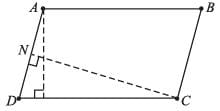AM = 7 cm, CN = 8 cm and AB = CD = 10 cm, Find AD.

Detailed Solution for Math Olympiad Test: Area of Parallelograms and Triangles- 2 - Question 2

Area of parallelogram
= AM × CD = AD × CN
⇒ 7 × 10 = AD × 8
⇒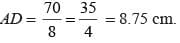Math Olympiad Test: Area of Parallelograms and Triangles- 2 - Question 3

### P, Q, R and S are the midpoints of DC, BC, AB and AD respectively. area of parallelogram PQRS is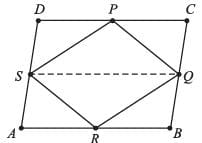Detailed Solution for Math Olympiad Test: Area of Parallelograms and Triangles- 2 - Question 3

Joining QS, it can be clearly seen that,
QS ∥ DC ∥ AB,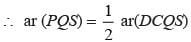[Area between QS and DC]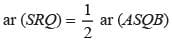[Area between QS and AB]
ar (PQS) + ar (SRQ) =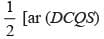+ ar (ASQB)]
ar (PQRS) = 1/2 ar (ABCD)

Math Olympiad Test: Area of Parallelograms and Triangles- 2 - Question 4

The median of a triangle divides is into two:

Detailed Solution for Math Olympiad Test: Area of Parallelograms and Triangles- 2 - Question 4

In ∆ABC, AD is the median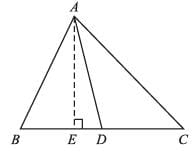Let AE ⊥ BC, then,
ar(∆ABD) = 1/2 × AE × BD,
ar(∆ADC) = 1/2 × AE × DC = 1/2 × AE × BD
∴  ar(∆ABD) = ar(∆ADC)

Math Olympiad Test: Area of Parallelograms and Triangles- 2 - Question 5

Ar(trapezium RQBC) =

Detailed Solution for Math Olympiad Test: Area of Parallelograms and Triangles- 2 - Question 5

Area of trapezium RQBC = ar(∆RBP) + ar(∆PQR) + ar(∆QPC)
= 3 × ar(∆PQR)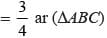Math Olympiad Test: Area of Parallelograms and Triangles- 2 - Question 6

If AD is median of ∆ABC and P is a point on AC such that ar(∆ADP) : ar(∆ABD) = 2 : 3, then ar(∆PDC) : ar(∆ABC) is

Detailed Solution for Math Olympiad Test: Area of Parallelograms and Triangles- 2 - Question 6

∵ AD median of ∆ABC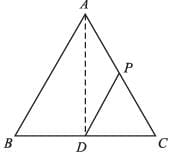∴ ar (ABD) = ar(ADC) = 1/2 ar(∆ABC) …(i)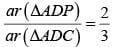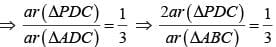∴ Required ratio =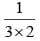= 1 : 6

Math Olympiad Test: Area of Parallelograms and Triangles- 2 - Question 7

ABCD is a trapezium in which AB ∥ CD and CD = 40 cm, and AB = 60 cm. If X and Y are, respectively, the mid points of AD and BC, then XY =

Detailed Solution for Math Olympiad Test: Area of Parallelograms and Triangles- 2 - Question 7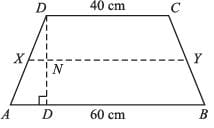∵ XY ∥ AB ∥ DC
[Let the length of XY be x cm]
∵ ar (DCYX) + ar (XYBA) = ar (ABCD)
∴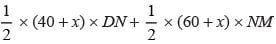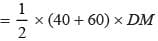…(i)
∵ DN = NM =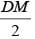∴ The equation (i) reduces to: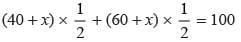⇒ 100 + 2x =  200
⇒ 2x = 100
⇒ x = 50 cm.

Math Olympiad Test: Area of Parallelograms and Triangles- 2 - Question 8

Area of trapezium, PQRS in the given figure is: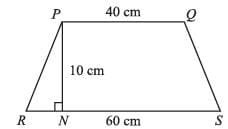Detailed Solution for Math Olympiad Test: Area of Parallelograms and Triangles- 2 - Question 8

Required area: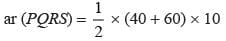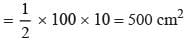Math Olympiad Test: Area of Parallelograms and Triangles- 2 - Question 9

Find the area of quadrilateral ABCD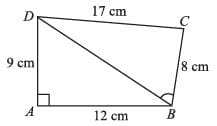Detailed Solution for Math Olympiad Test: Area of Parallelograms and Triangles- 2 - Question 9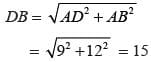ar(∆ADB) = 1/2 × 12 × 9 = 54 cm2
ar(∆DBC) = 1/2 × 15 × 8 = 60 cm2
∴ Total area = 54 + 60 = 114 cm2

Math Olympiad Test: Area of Parallelograms and Triangles- 2 - Question 10

ABCD is a parallelogram in which BC is produced to E such that CE = BC. AE intersects CD at F. If area ∆DFB = 3 cm2, area of parallelogram ABCD is:

Detailed Solution for Math Olympiad Test: Area of Parallelograms and Triangles- 2 - Question 10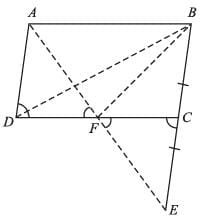ar(∆AFB) = 1/2 ar (parallelogram ABCD)
{areas between same parallels and same base}
⇒ ar (parallelogram ABCD) = 2 × ar(∆AFB) = 2 × ar(∆DCB) …(i)
In ∆ADF and ∆ECF
∠AFD = ∠EFC {vertically opposite angles}
∠ACF = ∠ADF {alternate angles}
BC = CE = AD
∴ ∆ADF ≅ ∆ECF {by AAS congruency}
∴ DF = CF
ar(parallelogram ABCD) = 2 × ar(∆DCB)
= 2 × 2 × (ar(∆DFB))
= 2 × 2 × 3
= 12 cm2

Math Olympiad Test: Area of Parallelograms and Triangles- 2 - Question 11

ABCD and FECG are parallelograms equal in area. If ar(∆AQE) = 12cm2, then ar(parallelogram FGBQ) =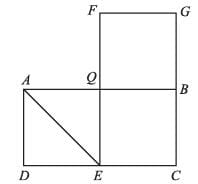Detailed Solution for Math Olympiad Test: Area of Parallelograms and Triangles- 2 - Question 11

ar(∆AQE) = 1/2ar (parallelogram AQED)
= 1/2 x 1/2 (ar(parallelogram ABCD))
= 1/4 × (ar(parallelogram FECG))
12 cm2 = 1/4 × ar(parallelogram FECG)
∴ ar(FECG) = 48 cm2
⇒ ar(FGQB) = 1/2 ar(FECG) = 48/2 = 24 cm2

Math Olympiad Test: Area of Parallelograms and Triangles- 2 - Question 12

ABCD is a parallelogram. P is the midpoint of AB. BD and CP intersect at Q such that QC : QP = 3 : 1. If the ar(∆PBQ) = 10 cm2, then area of parallelogram ABCD is:

Detailed Solution for Math Olympiad Test: Area of Parallelograms and Triangles- 2 - Question 12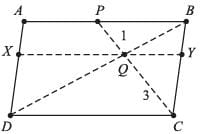ar(∆PQB) + ar(∆DQC) = 1/2 ar(parallelogram ABCD)
⇒ ar(parallelogram ABCD)
= 2 [ar(∆PQB) + ar(∆DQC)]
= 2 [10 cm2 + ar(∆DQC)]
ar(∆DQC) = (3 + 3 + 1) × ar(∆PQB)
= 7 × 10 = 70 cm2
∴ ar(parallelogram ABCD) = 2 × 80
= 160 cm2

Math Olympiad Test: Area of Parallelograms and Triangles- 2 - Question 13

M is a point on base QR of ∆PQR, and N is the midpoint of QR. NX is drawn parallel to MP at X. If ar(∆PQR) = 12cm2, then ar(∆XMR) =

Detailed Solution for Math Olympiad Test: Area of Parallelograms and Triangles- 2 - Question 13

Q ar(ΔXMR) = 1/2 ar(∆PQR)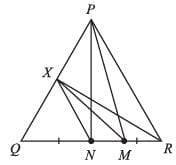∴ ar(∆XMR) = 1/2 × 12cm2 = 6 cm2

Math Olympiad Test: Area of Parallelograms and Triangles- 2 - Question 14

ABCD is a parallelogram, P and Q are the mid-points of BC and CD respectively, then, ar(∆APQ) = K (ar(ABCD)), K =

Detailed Solution for Math Olympiad Test: Area of Parallelograms and Triangles- 2 - Question 14

Let AB = CD = x, and BC = AD = y, AM = h1, BN = h2
ar (∆ADQ) = 1/2 × AM × DQ =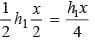ar (∆APB) =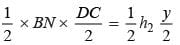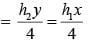ar (∆PQC) =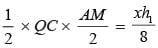ar (parallelogram ABCD) = AM × DC = BN × AD = h1x = h2y
∴ ar(∆AQC) = h1x –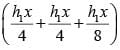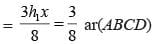Math Olympiad Test: Area of Parallelograms and Triangles- 2 - Question 15

∠AOB = 90°, AC = BC, OA = 12 cm and OC = 6.5 cm. The area (in cm2) of ∆AOB is: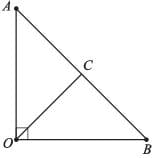Detailed Solution for Math Olympiad Test: Area of Parallelograms and Triangles- 2 - Question 15

OC = 6.5 cm,
∴ AC = BC = 6.5
[By similarly of ∆AOC and ∆BOC]
∴ AB = AC + BC = 2AC = 2 × 6.5 = 13 cm.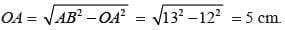∴ ar(∆AOB) = 1/2 × 5 × 12 = 30 cm2

## Mathematics Olympiad for Class 9

1 videos|43 tests
 Use Code STAYHOME200 and get INR 200 additional OFF Use Coupon Code
Information about Math Olympiad Test: Area of Parallelograms and Triangles- 2 Page
In this test you can find the Exam questions for Math Olympiad Test: Area of Parallelograms and Triangles- 2 solved & explained in the simplest way possible. Besides giving Questions and answers for Math Olympiad Test: Area of Parallelograms and Triangles- 2, EduRev gives you an ample number of Online tests for practice

## Mathematics Olympiad for Class 9

1 videos|43 tests